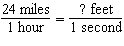Name:    Quiz 5.1-5.3

Multiple Choice
Identify the choice that best completes the statement or answers the question.

Write the ratio in simplest form.

1.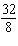a.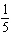c.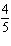b.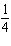d.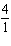2.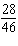a.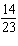c.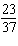b.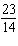d.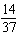3.

You use the punch recipe to create a larger batch that uses 6 liters of ginger ale. How many cans of pineapple juice should you use?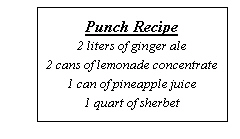a. 3 c. 12 b. 1 d. 6

Write a unit rate for the situation.

4.

\$4.50 for 9 people
 a.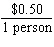c.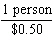b.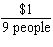d.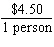5.

What are the units for the product?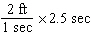a.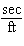c. ft b.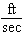d. sec

Find the distance.

6.

d  ?  , r = 41 m/sec, t = 5 sec
 a. 204 m/sec c. 205 m/sec b. 205 m d. 204 m

Find the speed.

7.

703 feet in 37 minutes
 a. 26,011 ft/min c. 23 ft/min b. 19 ft/min d. 703 ft/min

Find how far the object travels in the given amount of time.

8.

18 hours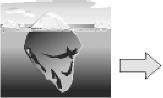Moves 2 meters every 3 hours.
 a. 36 m c. 12 m b. 27 m d. 8 m

9.

45 seconds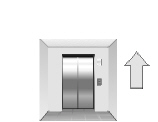Rises 5 stories every 9 seconds.
 a. 81 stories c. 24 stories b. 25 stories d. 225 stories

10.

42 seconds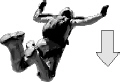Falls 2280 feet every 12 seconds.
 a. 8,000 ft c. 27,360 ft b. 7,955 ft d. 7,980 ft

Numeric Response

1.

You read 7 pages in 3 minutes. How many pages can you read in 6 hours?

Decide whether the rates are equivalent.

1.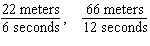Convert the unit rate.

2.